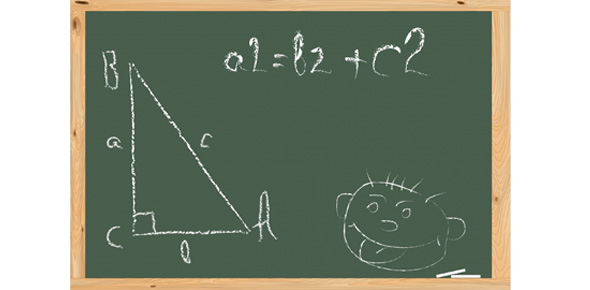# Pythagorean Theorem

4 Questions | Total Attempts: 70SettingsUse the Pythagorean Theorem to find the missing values. Note: All the following problems are lengths on a right triangle.

Related Topics
• 1.
If a = 5 and b = 12, c = ?
• A.

13

• B.

17

• C.

11

• D.

7

• 2.
If a = 7 and c = 25, b = ?
• A.

18

• B.

24

• C.

26

• D.

32

• 3.
If a = 9 and b = 10, c = ?   (Round answer to the tenth)
• A.

4.4

• B.

19

• C.

1

• D.

13.5

• 4.
If  b = 15 and c = 24, a = ?   (Round answer to the tenth)
• A.

28.3

• B.

18.7

• C.

9

• D.

39